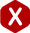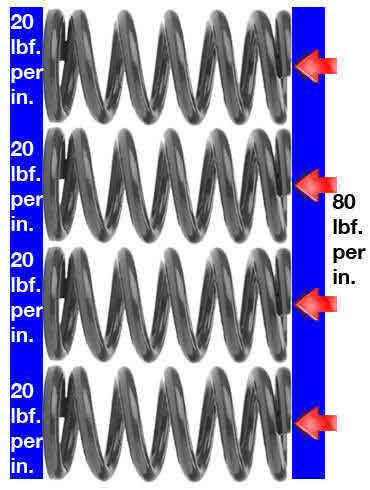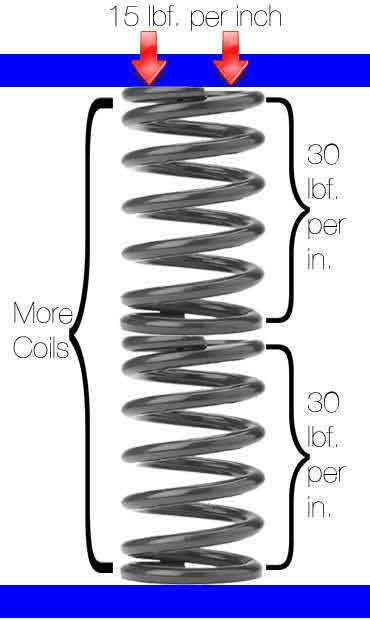<# Calculate Rate of Springs in Parallel and in Series

#### Calculate Rate of Springs in Parallel

Parallel spring rate is when you have two or more springs next to each other working together to support a structure. The spring rate of each spring is added together to calculate the equivalent constant force these springs will execute in your device or mechanism. Take a look at the examples provided below for a clearer explanation of why the spring rate of these springs is accumulative.

#### Example

You have four springs that have a 20 lbf/in (pounds of force per inch) rate. To calculate the spring rate in parallel you must add up the spring rates of all the springs as shown in the formula below.

Keq = k1 + k2 + k3 + k4

• Keq = Equivalent Rate
• k1 = Rate 1
• k2 = Rate 2
• k3 = Rate 3
• k4 = Rate 4
Keq = 20 + 20 + 20 + 20
Keq = 20(4)
Keq = 80 lbf/in (pounds of force per inch)#### Calculate Rate of Springs in Series

Springs in series are springs which are placed one on top of the other and are expected to work together in that form. In this case, the springs work as one so since there are more coils, the equivalent spring rate executed by these springs will be weaker than the spring rate of one of these springs alone. Take a look at the examples and diagram provided below for an explanation of how to calculate the rate of springs in series when the springs’ rates are either the same or different.

#### Example A. (Different Spring Rates)

You have two springs stacked one on top of the other. The rate of one of these springs is 20 lbf/in (pounds of force per inch) while the rate of the other spring stacked on top of this one is of 40 lbf/in. Multiply the rates together (k1 and k2) and divide the product by the sum of the rates as shown in the formula provided below. The resulting Keq is the new rate for the two springs in series.

Keq = (k1)(k2) / (k1 + k2)

• Keq = Equivalent Rate
• k1 = Rate of spring 1
• k2 = Rate of spring 2
Keq = (20)(40) / (20 + 40)
Keq = 800 / 60
Keq = 13.333 lbf/in (pounds of force per inch)

#### Example B. (Identical Spring Rates)

You have two identical springs with a spring rate of 30 lbf/in (pounds of force per inch). To calculate the rate these two provide together, simply multiply the rate by 1/2 (0.5). The formula for this calculation is also provided below. The resulting Keq is the new rate for the two springs in series.

Keq = ½ (k)

• Keq = Equivalent Rate
• k = rate
Keq = ½ (30)
Keq = 15 lbf/in (pounds of force per inch)